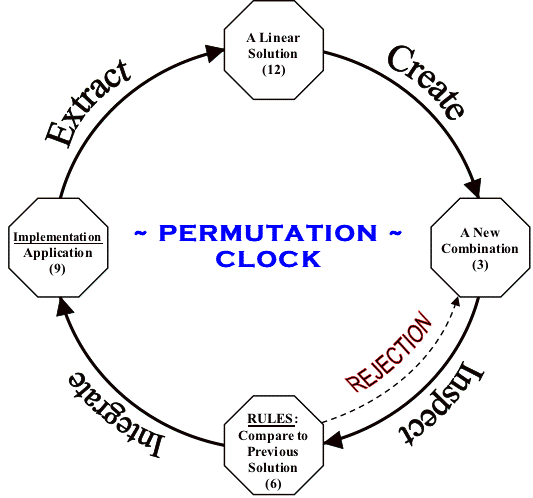The Countdown Meta-Permutation Class: Presented as Example_05

"Is there a function or class that returns just two index values (i, j) such that SWAP(a[i], a[j]) always produces a unique combination within an array a[]?" ~ Dr. Elaine R. Milito (previously unsolved)

Presented below is the C++ algorithm which answered Dr. Milito's question proposed sometime after the Fall of 1986. Using a target array of size N, two distinct variables (j, i) can be accessed for all head transpositions as well as two distinct variables (q, r) for all tail transpositions. Given two target arrays called h[N], t[N] and the Meta-permutation object s(N), SWAP(h[s.j], h[s.i]) is valid for all head combinations whereas SWAP(t[s.q], t[s.r]) is valid for all tail combinations provided that all SWAP calls are followed by a single s.next() call to calculate four new index values.

Using a nested while loop in the countdown QuickPerm algorithm clearly lead to the development of a Meta-permutation class (below). In contrast, replacing the nested while loop with a conditional if-else statement as described in the counting QuickPerm algorithm causes the Meta-permutation class to "skip" index calculations due to an obvious dependency on the outer loop. Although this conditional substitution can be made regardless of the counting process utilized, the Meta-Permutation Class precludes such a substitution in order to return valid indices.

Example_05 efficiently combines the four previous examples by utilizing a single Meta-Permutation Class aptly named MetaPerm. Here's the Meta-Permutation Class written in C++ for your review:

MetaPerm Class - Combining the Four Previous Examples

MetaPerm Function to Display Permutations (Optional)
 // NOTICE: Copyright 1991-2008, Phillip Paul Fuchs                                           void display(unsigned int *a) { for(unsigned int x = 0; x < N; x++) printf("%d ",a[x]); printf("\n"); // getch(); // Remove comment for "Press any key to continue" } // display()[]

[] | [] | [] | [] | [MetaPerm]
[] | []
[] | [] | []
[]